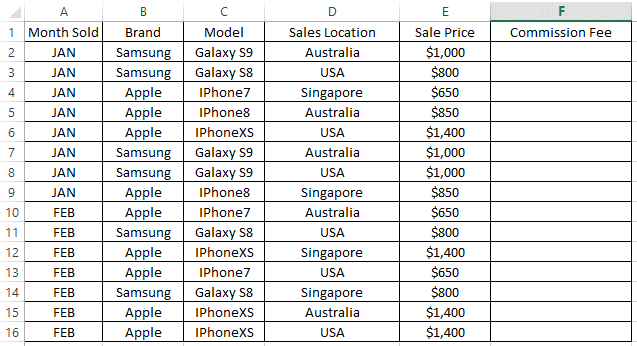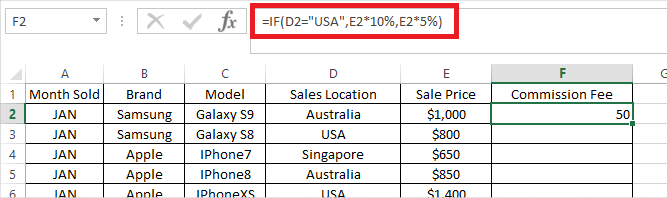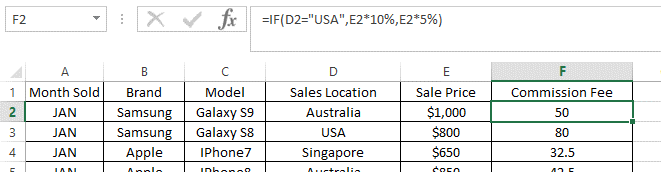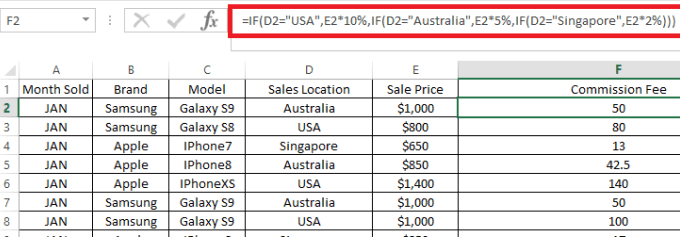# Computer help - file server

[ Blog ] - [ File Server ] - [ Удаленная компьютерная помощь ]

One Excel function that I use quite a bit in my formulas is the IF function. The IF function is used to test a logical condition and produce two different results depending on whether the logical condition returns TRUE or FALSE.

Let’s use the mobile phone sales table below as an example. You can download the example file here.IF Function with Single Condition

Consider a scenario where you need to calculate the Commission Fee for each sales row, depending on where the sales was made (Column D). If the sales was made in the USA, the Commission Fee is 10%, otherwise the remaining locations will have Commission Fee of 5%.

The first formula that you need to enter on Cell F2 is as shown below: =IF(D2="USA", E2*10%, E2*5%)Formula breakdown: =IF( – The “=” indicates the beginning of a formula in the cell and IF is the excel function that we are using. D2=”USA” – Logical test that we perform (i.e. if data in column D2 is USA).  E2*10% – Result that will be returned by the formula if the initial logical test results in TRUE (i.e. value in column D2 is USA). E2*5% – Result that will be returned by the formula if the initial logical test results in FALSE (i.e. value in column D2 is NOT USA). ) – Closing bracket indicating the end of the formula.

Then you can copy down the formula from Cell F2 to the rest of the rows in Column F and it will calculate the Commission Fee for each line, either by 10% or 5% dependent on whether the IF logical test returns TRUE or FALSE on each row.IF Function with Multiple Conditions

What if the rules were a bit more complicated where you need to test for more than one logical condition with different results being returned for each condition?

Excel has an answer to this! We can combine multiple IF functions within the same cell, which is sometimes known as a Nested IF.

Consider a similar scenario where the Commissions are different for each Sales Location as below: USA 10% Australia 5% Singapore 2%

In Cell F2 (which later will be copied to the rest of the rows in the same column F), enter the formula as follow: =IF(D2="USA",E2*10%,IF(D2="Australia",E2*5%,E2*2%))

Formula breakdown: =IF( – Beginning of the formula using an IF statement D2=”USA” – First logical test that we perform (i.e. if data in column D2 is USA).  E2*10% – Result that will be returned by the formula if the initial logical test results in TRUE (i.e. value in column D2 is USA). IF(D2=”Australia”,E2*5%,E2*2%) – second Excel IF statement that will be assessed if the initial logical test resulted in FALSE (i.e. value in column D2 is NOT USA). This is a similar syntax of “IF Function with Single Condition” discussed earlier in this article where if value on Cell D2 is Australia, the result of E2*5% will be returned. Otherwise, if the value is not Australia, the function will return result of E2*2%. ) – Closing bracket indicating the end of the formula for the first IF function.

As Excel will assess the formula from the left to the right, when a logical test is met (e.g. D2=“USA”, the function will stop and return the result, ignoring any further logical test after (e.g. D2=“Australia”.)

So if the first logical test returns FALSE (i.e. location is not USA), it will continue to assess the second logical test. If the second logical test returns FALSE as well (i.e. location is not Australia), we do not need to test further as we know the only possible value on Cell D2 is Singapore hence it should return a result of E2*2%.

If you prefer for clarity, you can add the third logical test IF(D2=”Singapore”, “value if TRUE” , “value if FALSE”). Therefore, the full extended formula is as shown below: =IF(D2="USA",E2*10%,IF(D2="Australia",E2*5%,IF(D2="Singapore",E2*2%)))As mentioned earlier, the above will return the same result as the initial formula that we had. =IF(D2="USA",E2*10%,IF(D2="Australia",E2*5%,E2*2%)) Quick Tips For every single IF( function, there needs to be an opening and closing round bracket. When there are three IF functions as per one of the examples above, the formula will need three closing brackets “)))”, each marking the ending of a corresponding opening IF( statement. If we do not specify the second outcome of the logical test (when the logical test resulted in FALSE), the default value assigned by Excel will be the text “FALSE”. So formula =IF(D2=”USA”,E2*10%) will return the text “FALSE” if D2 is not “USA”. If you have several different logical tests each with its own different outcome, you can combine/nest the IF function multiple times, one after another, similar to the example above.

SETUP UA COMPUTER BLOG